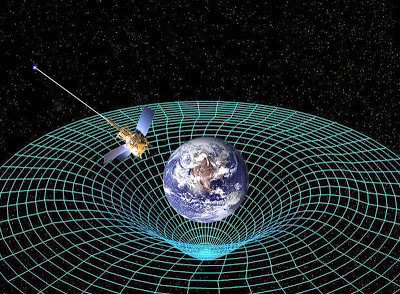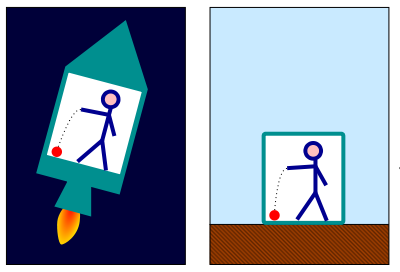### A List of Dubious Concepts in General RelativityWater curvature and a metallic ball
By Johny Jagannath

1. Matter curves a medium [also known as spacetime] that is not made out of a substance. But simple logic tells us that if a substance and non-substance collide, they will freely penetrate each other. Therefore the curving shown below [in the picture with the Earth] must be an action-at-a-distance phenomenon.

The action-at-a-distance nature of this curving of the medium can be easily understood with the help of water and a metallic ball. Imagine a metallic ball is placed in a bucket of water. The water curves around the metallic ball. This curvature is not action-at-a-distance since the water molecules makes contact with the ball where it curves. [See the figure above]. But imagine that the water curves around the metallic ball in such a way that it does not physically touch the metallic ball? Isn’t this curvature action-at-a-distance [as shown in the second bucket above]? It is. General Relativity’s curvature [of spacetime] is similar to water curving around the metallic ball without making a physical contact, as seen in the figure below. [See the white lines.]Spacetime curvature
Further more: two objects curve the medium in such a way that the two objects automatically meet each other, sliding down the curvature of the medium. Again there is no hint of mechanics going on here. Simple assertions and action-at-a-distance.

This is the same as Newton’s theory of gravity. Newton postulated that two objects separated by a distance, automatically meet each other. Of course this is action-at-a-distance. But General Relativity is no different, it is mathematically equivalent to Newton’s theory of Gravity. [We will look at the precession of the planetary orbits a little later in this post.]

2. The speed of Gravity is assumed to be finite [equal to speed of light], in General Relativity. But in Newton’s theory of gravity the speed of gravity is instantaneous. The reason it is assumed to be instantaneous is because a finite propagation delay would cause the planetary orbits to become unstable. We know that light from our Sun takes 8 minutes to reach the Earth. If the speed of gravity were the same as the speed of light, our Earth would not feel any effects of gravity for 8 minutes during which time, the Earth would simply veer off of its regular orbital path and cause a visibly unstable orbit for astronomers to see. This has never been observed. Therefore, why make an assumption that is provably wrong?3. The Equivalence Principle is a dubious approximation and mathematically incorrect when there is a frame to frame comparison being made. Here the frames are a room and a spaceship. Gravity depends on distance and changes at every point inside a room [on the Earth as measured from the center of the Earth]. Therefore a spaceship accelerating at 1g is not mathematically or physically equivalent to room on the Earth. Since gravity depends on distance, the amount of gravity experienced at the feet is higher than what is experienced at the head. Here’s how Wikipedia describes it…

“So the original equivalence principle, as described by Einstein, concluded that free-fall and inertial motion were physically equivalent. This form of the equivalence principle can be stated as follows. An observer in a windowless room cannot distinguish between being on the surface of the Earth, and being in a spaceship in deep space accelerating at 1g. This is not strictly true, because massive bodies give rise to tidal effects (caused by variations in the strength and direction of the gravitational field) which are absent from an accelerating spaceship in deep space.”

4. The precession of the planetary orbits is explained mathematically via a central force, a concept that Newton developed for his own theory. But the formula that Newton and others developed were not accurate. In one peculiar case, Paul Gerber derived a formula [for the precession of planet Mercury] that was mathematically identical to Einstein’s, years before Einstein did. Therefore the explanation of the precession of the planetary orbits is to me a mathematical problem than a problem of physics. Note that by using a central force one is giving up the inverse square law framework.

My theory on the other hand, provides a physical/substantial/material account of the phenomenon of gravity and it successfully explains why gravity does what it does via the laws of mechanics without resorting to action-at-a-distance or by giving up the inverse square law framework.

I call my theory, “An Archimedean Screw Interpretation of Gravity”. The evolution of an Archimedean Screw is explained in the Chapter titled, “The Story Of Our Universe”, which has experimental proof to support the hypothesis.